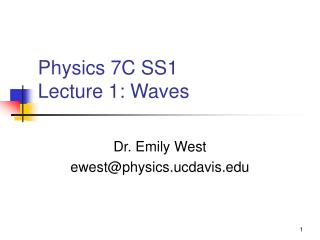DownloadDownload PresentationPhysics 7C SS1 Lecture 1: Waves

# Physics 7C SS1 Lecture 1: Waves

Download Presentation## Physics 7C SS1 Lecture 1: Waves

- - - - - - - - - - - - - - - - - - - - - - - - - - - E N D - - - - - - - - - - - - - - - - - - - - - - - - - - -
##### Presentation Transcript

1. Physics 7C SS1Lecture 1: Waves Dr. Emily West ewest@physics.ucdavis.edu

2. Agenda • Logistics: Syllabus and Web Page • Clicker Setup • Course Overview--the models of 7C • Simple Harmonic Motion Review • Wave Introduction

3. Course webpage • http://physics.ucdavis.edu/physics7

4. Clicker Setup: Joining Class • Turn on your clicker; it scans for classes • If it doesn’t scan automatically, hit enter (green button) • Find 09SS1PHY7C; hit enter • Display reads “ANS: 09SS1PHY7C;” hit enter • When you’ve successfully joined, display reads “ANS: wait for Q”

5. Clicker Setup: Student ID • Turn on your clicker • Press * to access Setup Menu • Scroll to find “ID;” hit Enter (green button) • Enter your Student ID so I can give you credit; hit enter

6. Check Clicker Setup When did you take 7B? • Spring 09 • Winter 09 • Fall 09 or earlier • Didn’t take 7B (but took 7A) • I haven’t taken any 7-series classes

7. Course Overview:Models of 7C • Wave Model • Waves in 1, 2, and 3 dimensions • Interference • Ray Model • Reflection & Refraction • Lenses • Field Model • Electricity • Magnetism

8. Simple Harmonic Motion Review How can we describe the motion of oscillating objects?

9. Simple Harmonic Motion (SHM) Constructs • Amplitude: maximum distance from equilibrium • Period (or frequency): amount of time required to complete a cycle (or number of cycles per unit time) • Equilibrium: position at which all forces acting on the object sum to zero. • Displacement: position of the object relative to equilibrium • Restoring Force: a force that acts on an object that tends move it toward equilibrium • Defining SHM: The restoring force is proportional to the displacement. • Anything missing?

10. Mathematics of SHM y y=Displacement A=Amplitude T=Period t

11. Mathematics of SHM y=Displacement A=Amplitude T=Period y 1 6 In this image, which numbers, if any, show… Displacement? 4 t 3 2 5

12. Mathematics of SHM y=Displacement A=Amplitude T=Period y 1 6 In this image, which numbers, if any, show… 4 t 3 2 Amplitude? 5

13. Mathematics of SHM y=Displacement A=Amplitude T=Period y 1 6 In this image, which numbers, if any, show… 4 t 3 2 5 Period?

14. Mathematics of SHM y y=Displacement A=Amplitude T=Period A In this image, what is the mass doing at t=0 seconds? t T A Is the equation correct as is? T y(t) = A sin (2p t/T)???

15. Mathematics of SHM y y=Displacement A=Amplitude T=Period T f=phase t In this image, what is the mass doing at t=0 seconds? A In this image, what value does fhave? y(t) = A sin (2p t/T + f) y(t) = A sin (2p t/T)?

16. Introduction to Waves

17. Questions to consider about waves… • What new constructs are needed to describe waves? • What constructs can I control when I start the wave? • What constructs are set by the medium that carries the wave? • What moves from one end of the machine to the other?

18. Definition of Wave • Waves: A transfer of energy without bringing along the mass. • Particles in the medium get disturbed and collide, but they stay oscillating about one position; they don’t travel with the wave. • The medium does not effectively move. The disturbance advances, that’s the wave.

19. A vibrating object acts as the source of the wave. As a speaker moves through a cycle, it fixes the period and frequency. • A = Amplitude, controlled by what starts the wave • T = Period, how long to get back to where it started  = Wavelength, repeat distance in space T = where f is the frequency Velocity v =  f (note meters per second)